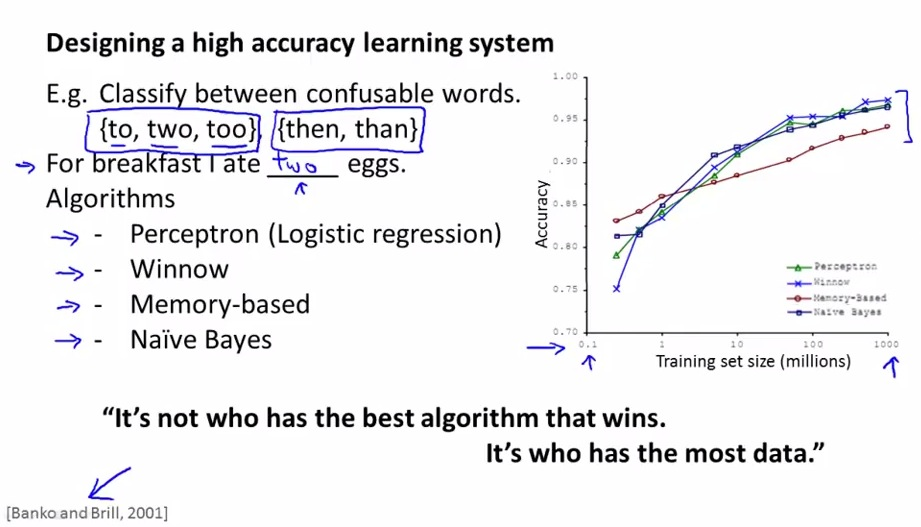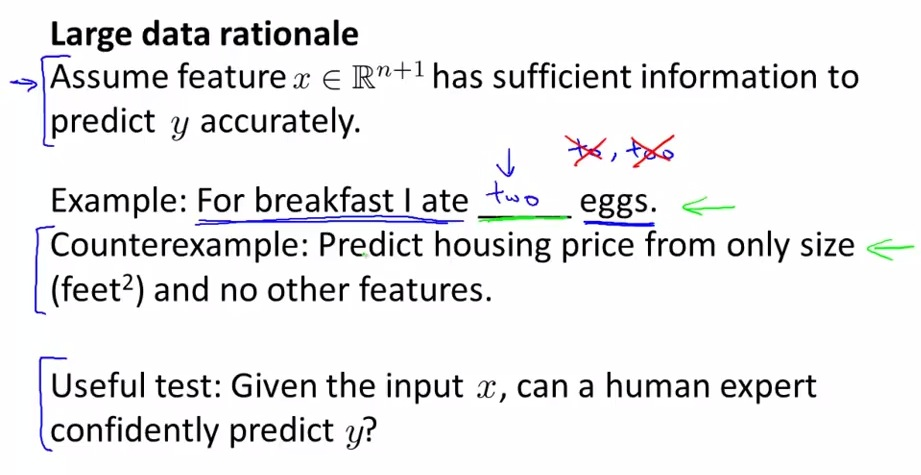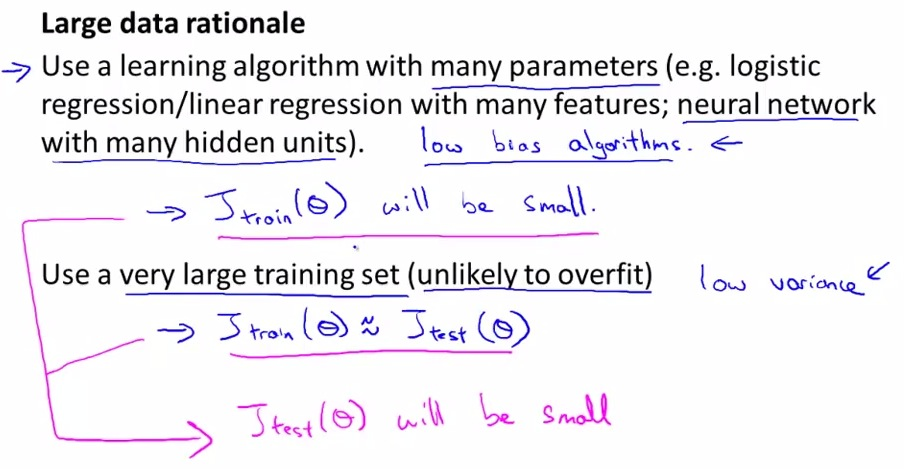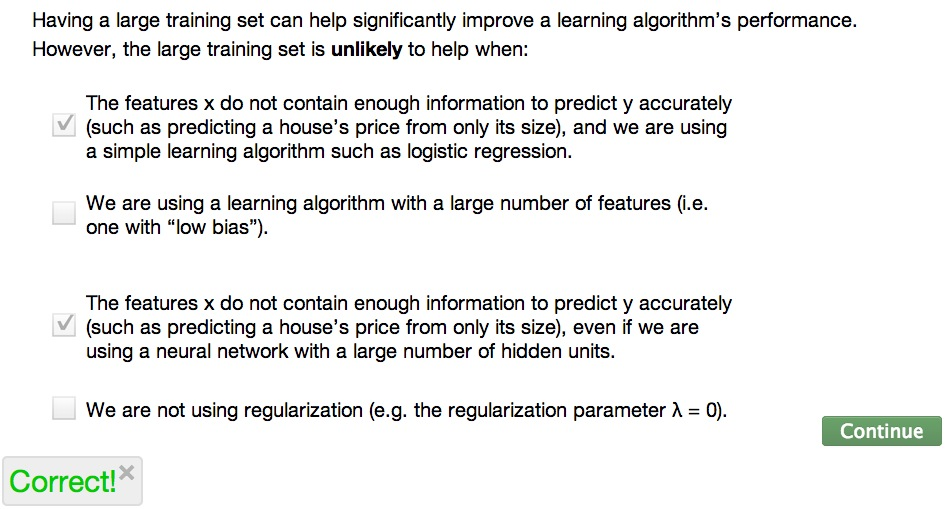• Previous : Evaluation matrix
• Earlier, we talked about not bluntly gather as much data as we can, as it not always the solution.
• For particular condition, learning algorithm can be more effective by taking more data.
• This lesson is about to explore more about the particular condition• Two scientist doing research on confusable words.
• They doing various learning algorithms and test its accuracy
• Winnow more or less logistic regression. With memory-based now not much used.
• More data with any algorithm eventually showing the same result, even "the inferior algorithm" will be catch up to most effective learning algorithm.
• Which led to statements above.
• Is it true? When is it true? When is it not true?• They believe if we have sufficient information we can predict the output correctly
• Then ask this? Can we make a better prediction if we have one feature?
• Sometimes, very difficult to predict the output if we have one feature(particularly in house example).
• Try to rely on human expert to see whether they can predict the value output given one feature. For confusable words, yes. But for house example, even realtor can't predict the price given only size of the house information.
• Next let's discussed the assumption as mentioned above is true.• Above are examples of many algorithms that can include very complete function/ incorporate many features. Then that means the algorithm has low bias.
• And given lots of data, the learning algorithm is likely less overfit
• Incorporate these both method we can have low bias and low variance.
• And if Jtrain is low, and Jtrain approximately equal to Jtest, then we will have high performance learning algorithm.• Checkbox 2 and 4 is wrong. Because it is the cases where we have overfit problem. so increasing training set(very large) will be a huge help.
• Checkbox 1 and 3 is correct. Because we don' have enough features, even large training set will not give enough help to increase learning algorithm to predict the correct value

• In summary , having lots of data, and lots of features will give us a powerful learning algorithm.
• Key test to observe:
• Try with human expert to predict the output value (y value). Try to see if the feature given are possible for human to solve.
• Next, if we have lots of parameters and lots of training sets, then we will have significantly better learning algorithm